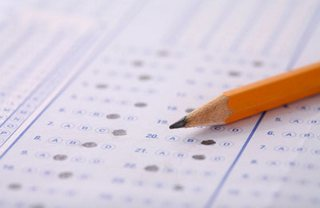# MCQs with keyIn this one PDF, MCQs of all chapters of FSc Part2 are given. There are seven chapters. Keys of MCQs is starting from page 51.

• A function $I(x)=x$ is called
• (A) A linear function
• (B) An identity function
• (D) A cubic functions
• $\frac{d}{dx} \tan 3x =$….
• (A) $3\sec^2 3x$
• (B) $\frac{1}{3}\sec^2 3x$
• (C) $\cot 3x$
• (D) $\sec^2 x$
• If $y=f(x)$, then differential of $y$ is
• (A) $dy=f'(x)$
• (B) $dy=f'(x) dx$
• (C) $dy=f(x)$
• (D) $\frac{dy}{dx}$
• If $x<0$, $y<0$, then the point $P(x,y)$ lies in the quadrant
• (A) I
• (B) II
• (C) III
• (D) IV
• The solution of $ax+by<c$ is
• (A) closed half plane
• (B) open half plane
• (C) circle
• (D) parabola
• The locus of the revolving line with one end fixed and other end on the circumference of the circle is called
• (A) a sphere
• (B) a circle
• (C) a cone
• (D) a conic
• The vector whose magnitude is $1$ is called
• (A) null vector
• (B) unit vector
• (C) free vector
• (D) scalar

These are just samples. PDF file of all the MCQs can be downloaded from the link given below.

• fsc/fsc_part_2_mcqs/mcqs_with_key